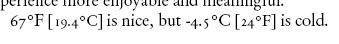# Converting Temperatures in LaTeX

I’m working on a publication that includes a lot of temperatures, and I want conversions of those temperatures to be automatically inserted into the text in a consistent manner and without having to convert them by hand. Michael Mehlich’s excellent fp-package provides some useful functionality for accomplishing this.

`\usepackage{fp} `

I’ve defined a tempc and tempf command that accept a single value and output a nicely formatted conversion. Using “\tempf{67} is nice, but\tempc{-4.5} is cold.” produces the output below. Note that the text inside the brackets is set a bit smaller. Conversions from Fahrenheit to Celsius always get one digit after the decimal point. This example uses text figures (The vertical positions of the numerals vary to make them more readable on a line.) as opposed to line figures.LaTeX Temperature Conversion Example

Here’s the code:

```% Temperatures... \newcommand{\tempc}{\FPmul{\x}{#1}{1.8}\FPadd{\x}{\x}{32}\FPround{\x}{\x}{0} #1\textcelsius\ [{\small\x\textdegree F}]} \newcommand{\tempf}{\FPsub{\x}{#1}{32}\FPmul{\x}{\x}{.5555555}\FPround{\x}{\x}{1} #1\textdegree F [{\small\x\textcelsius{}}]} ```

Tags: , ,

This site uses Akismet to reduce spam. Learn how your comment data is processed.